# 3. This example hopes to illustrate why the vector spaces the linear transformation are defined o...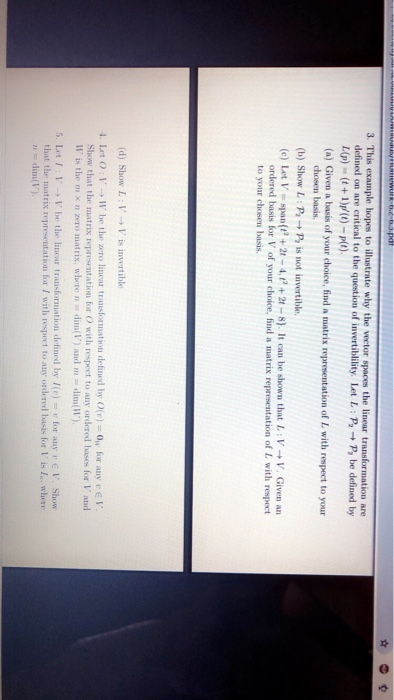3. This example hopes to illustrate why the vector spaces the linear transformation are defined on are critical to the question of invertibility. Let L : → p, be defined by L(p)(t+1)p(t)-plt). (a) Given a basis of your choice, find a matrix representation of I with respect to your chosen basis (b) Show L: P+P is not invertible (e) Let V-span+21-4,+2t-8). It can be shown that L VV. Given an ordered basis for V of your choice, find a matrix representation of L with respect to your chosen basis. (d) Show L : V → V is invertible ·Let O : 1. → 11. be the zero linear transfrination defined by O(r)-O" for any r e l Show that the matrix representation for O with respect to any ordered bases for V' and ll' is the m x n zero matrix, where n-dim(V) and m = dim(W) 5. Let 1 : V → V le the linear transformation defined by I(e) r for any r € 1". Show that the matrix represeutation for I with respect to any ordered basis for V is I where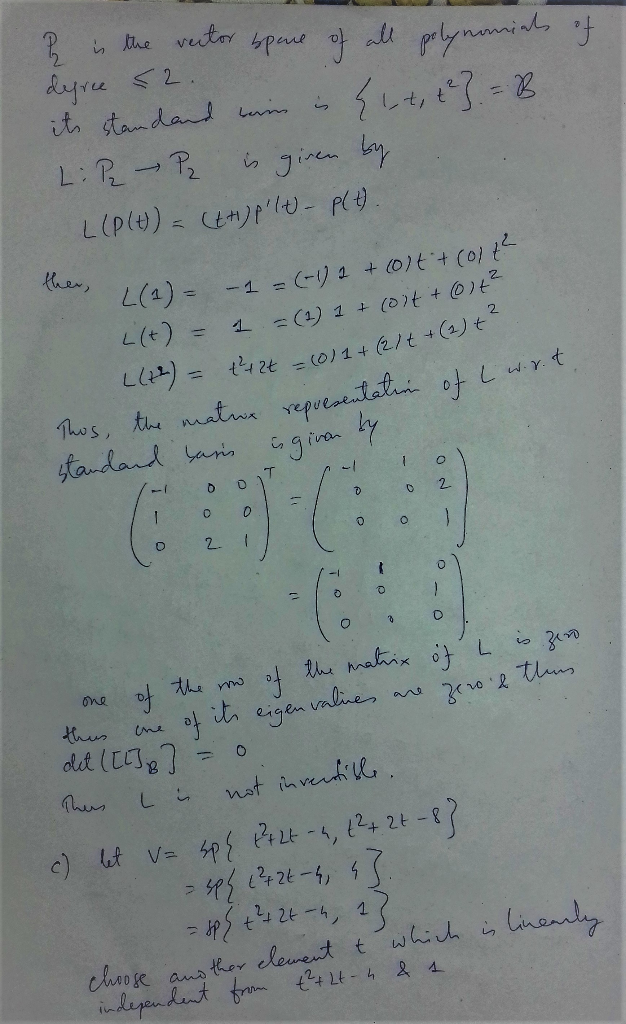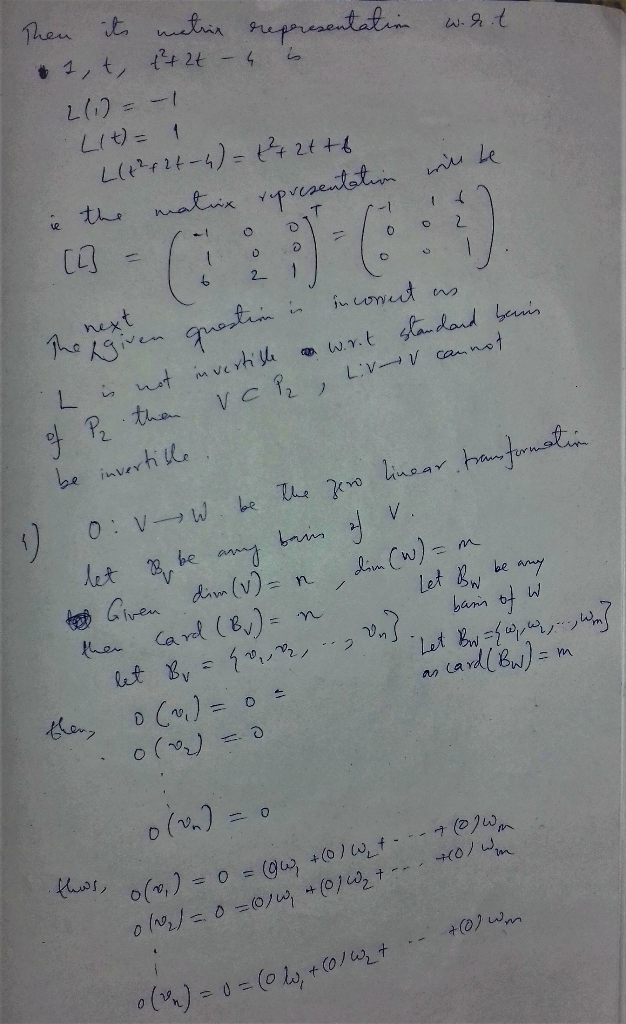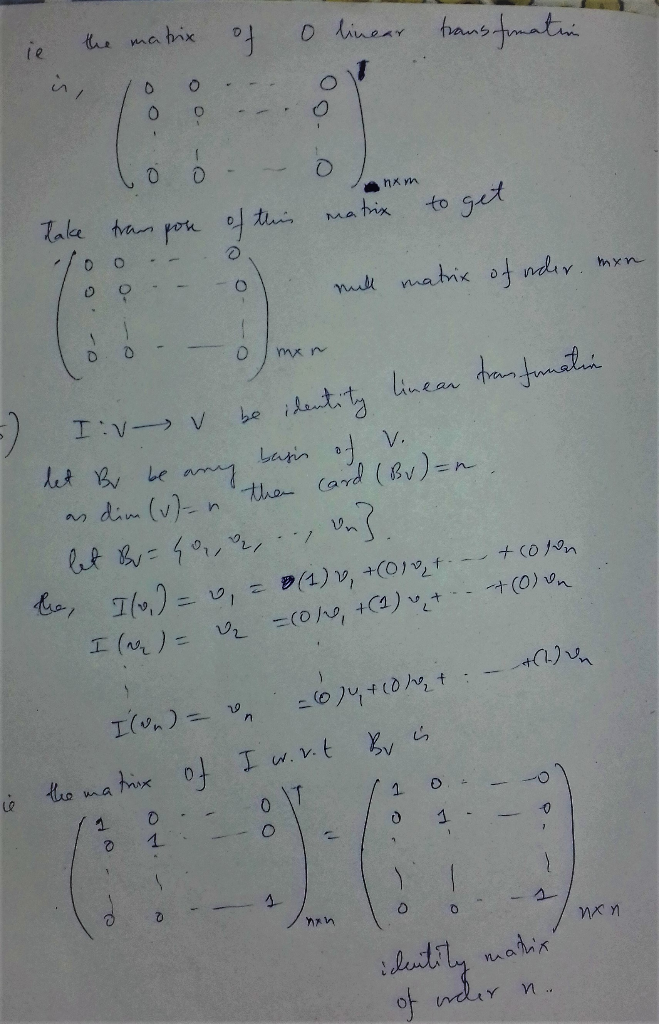#### Earn Coin

Coins can be redeemed for fabulous gifts.

Similar Homework Help Questions
• ### How was the linear transformation of b1 and b2 were applied (L(b1) , L(b2))? NOTE: b1=(1,1)^T , b2=(-1,1)^T Linear Transformations EXAMPLE 4 Let L be a linear transformation mapping R? into itself an...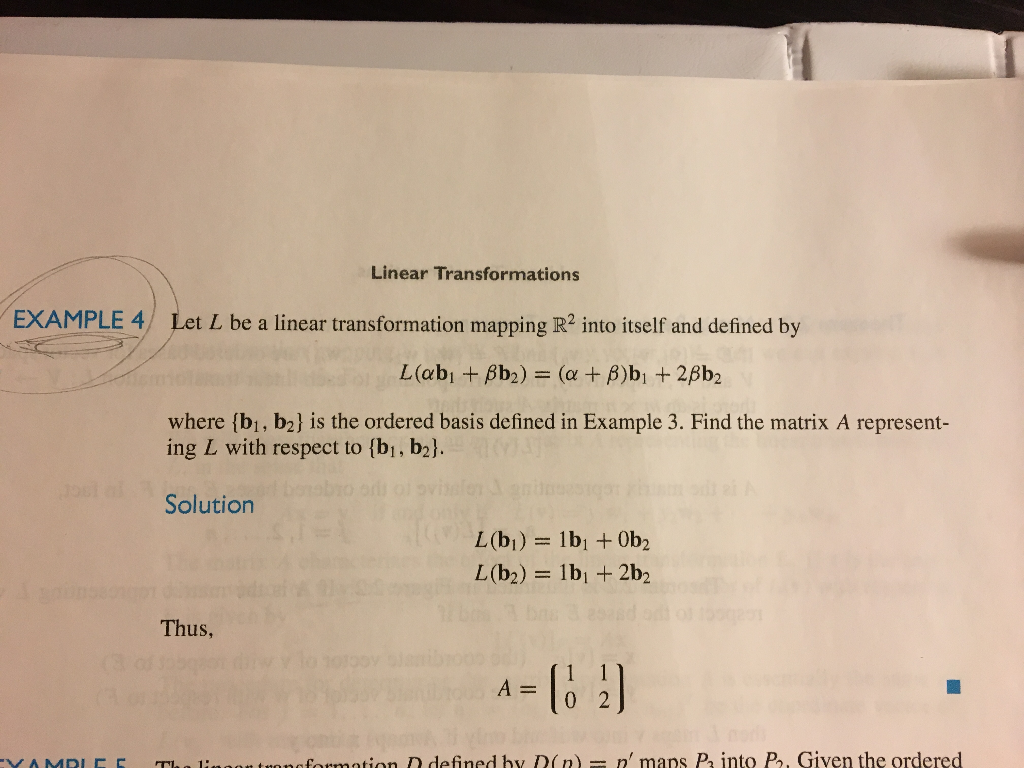How was the linear transformation of b1 and b2 were applied (L(b1) , L(b2))? NOTE: b1=(1,1)^T , b2=(-1,1)^T Linear Transformations EXAMPLE 4 Let L be a linear transformation mapping R? into itself and defined by where (bi, b2] is the ordered basis defined in Example 3. Find the matrix A represent- ing L with respect to [bi, b2l Solution Thus, A0 2 onofosmation D defined by D(n n' maps P into P, Given the ordered Linear Transformations EXAMPLE 4 Let...

• ### 4) The linear transformation L defined by L(p(x)) = p'(x)+p(0) maps Pinto P. a) Find the...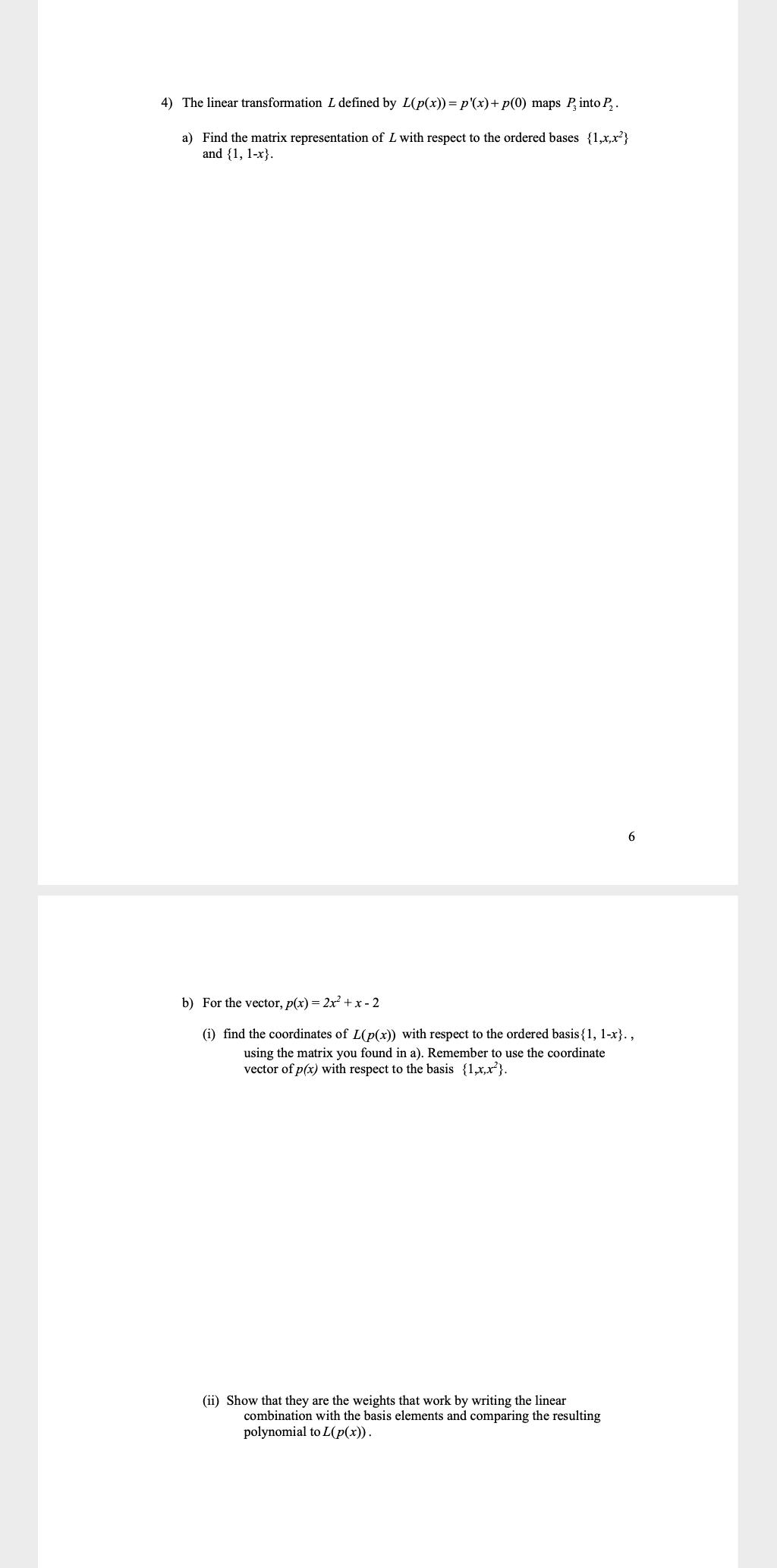4) The linear transformation L defined by L(p(x)) = p'(x)+p(0) maps Pinto P. a) Find the matrix representation of L with respect to the ordered bases {1,x,x} and {1, 1-x}. 6 b) For the vector, p(x) = 2x + x - 2 (i) find the coordinates of L(p(x)) with respect to the ordered basis{1, 1-x}. , using the matrix you found in a). Remember to use the coordinate vector of p(x) with respect to the basis {1,x,x"}. (ii) Show that...

• ### 4) The linear transformation L defined by L(p(x)) = p'(x)+ p(0) maps P, into P. a)...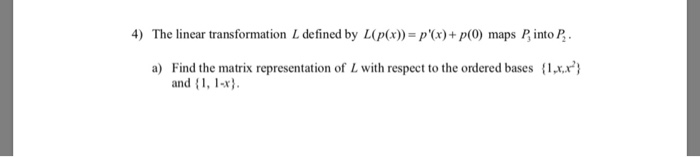4) The linear transformation L defined by L(p(x)) = p'(x)+ p(0) maps P, into P. a) Find the matrix representation of L with respect to the ordered bases {1xx.x"} and {1, 1-x} b) For the vector, p(x) = 2x2 + x-2 () find the coordinates of L(p(x)) with respect to the ordered basis {1, 1-x}., using the matrix you found in a). Remember to use the coordinate vector of p(x) with respect to the basis {1xx"}. (ii) Show that they...

• ### 4) The linear transformation L defined by L(p(x)) = p(x)+p(0) maps Pinto P. a) Find the...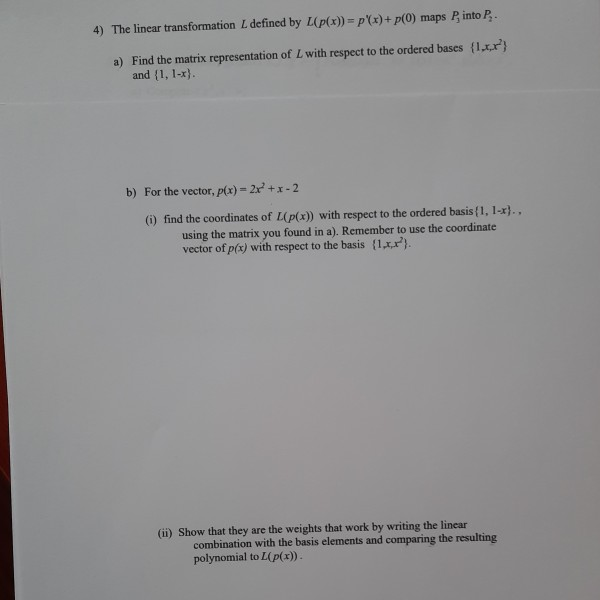4) The linear transformation L defined by L(p(x)) = p(x)+p(0) maps Pinto P. a) Find the matrix representation of L with respect to the ordered bases l_r"} and {1, 1-x). b) For the vector, p(x) = 2x' +1-2 () find the coordinates of L(p(x)) with respect to the ordered basis{1, 1-x), using the matrix you found in a). Remember to use the coordinate vector of p(x) with respect to the basis {1x2). (ii) Show that they are the weights that...

• ### Font Styles Paragraph Definition 1: Given La linear transformation from a vector space V into itself,...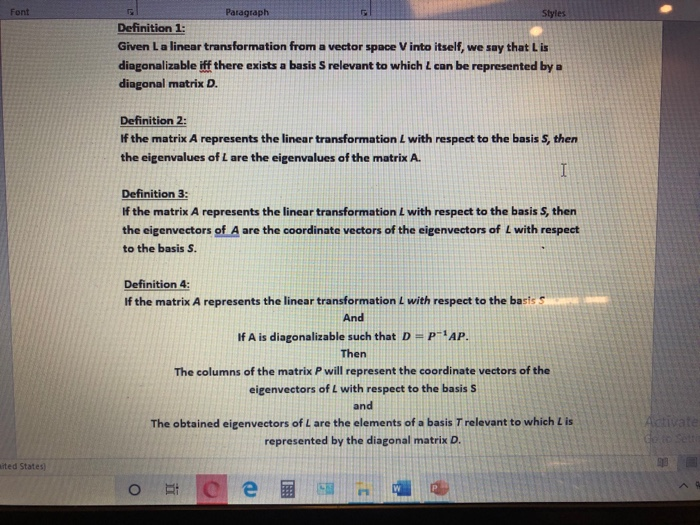Font Styles Paragraph Definition 1: Given La linear transformation from a vector space V into itself, we say that is diagonalizable iff there exists a basis S relevant to which can be represented by a diagonal matrix D. Definition 2: If the matrix A represents the linear transformation L with respect to the basis S, then the eigenvalues of L are the eigenvalues of the matrix A. I Definition 3: If the matrix A represents the linear transformation L with...

• ### Let T R3 R4 be the linear transformation defined by T(π1, Ο2, 73) - ( 3α1...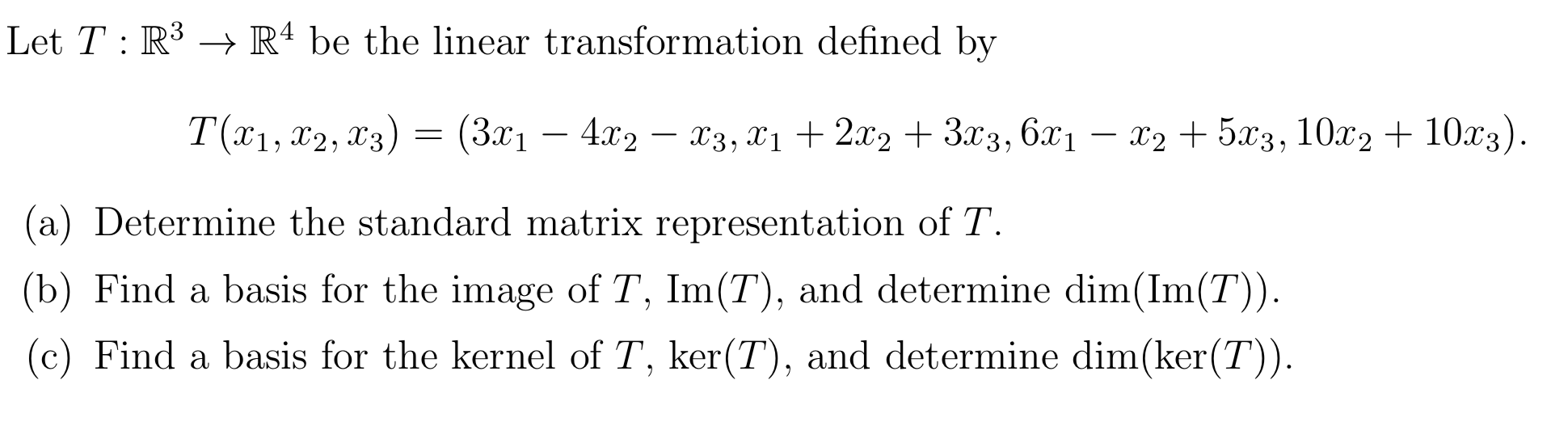Let T R3 R4 be the linear transformation defined by T(π1, Ο2, 73) - ( 3α1 -4 , X3, 12.x2 3.x3, 6x1-25x3, 10x2 + 10x3) (a) Determine the standard matrix representation of T (b) Find a basis for the image of T, Im(T), and determine dim(Im(T)) (c) Find a basis for the kernel of T, ker(T), and determine dim(ker(T))

• ### 11. =(7.5), #,(-3,-1) 2) Let = (1.-5). v. =(-2,2) and let L be a linear operator...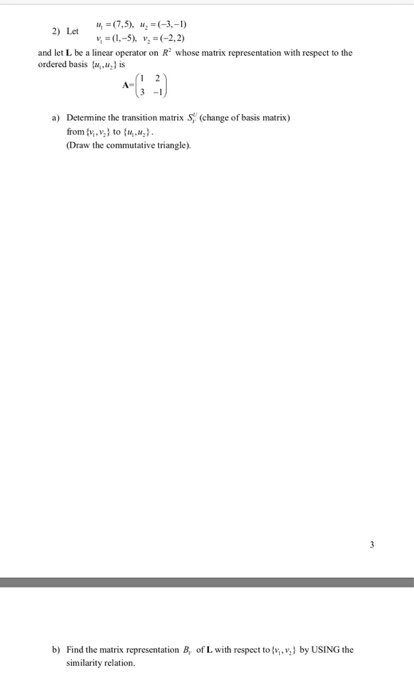11. =(7.5), #,(-3,-1) 2) Let = (1.-5). v. =(-2,2) and let L be a linear operator on R whose matrix representation with respect to the ordered basis . is a) Determine the transition matrix (change of basis matrix) from, v,to (1) (Draw the commutative triangle). 3 b) Find the matrix representation B, of L with respect to ,v} by USING the similarity relation

• ### Let x = [xı x2 x3], and let TER → R be the linear transformation defined...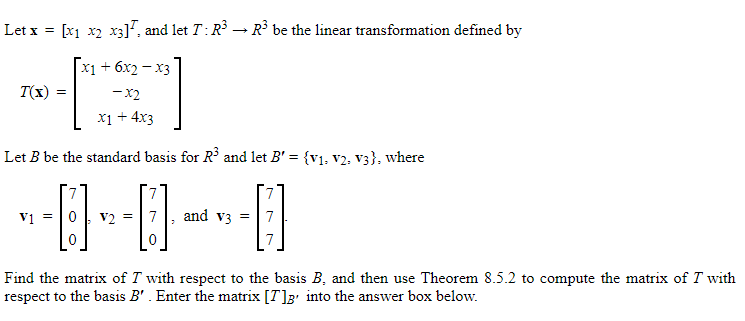Let x = [xı x2 x3], and let TER → R be the linear transformation defined by T() = x1 + 6x2 – x3 -X2 X1 + 4x3 Let B be the standard basis for R2 and let B' = {V1, V2, V3}, where 7 7 and v3 = 7 V1 V2 [] --[] 0 Find the matrix of I with respect to the basis B. and then use Theorem 8.5.2 to compute the matrix of T with respect to...

• ### 1 6) Let L: R→ R* be defined as L(A) = A. (1 2) (1996.)A OC...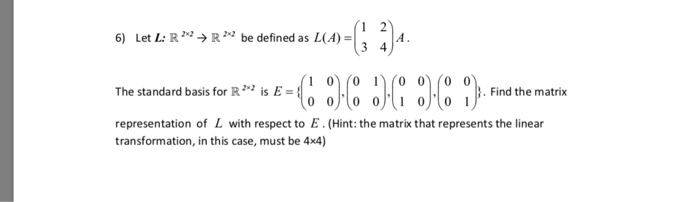1 6) Let L: R→ R* be defined as L(A) = A. (1 2) (1996.)A OC :) The standard basis for R2 is E = { Find the matrix representation of L with respect to E. (Hint: the matrix that represents the linear transformation, in this case, must be 4x4)

• ### Q4. Let L: R2 + Rº be a transformation defined by L (0-2 [3u2 – U1...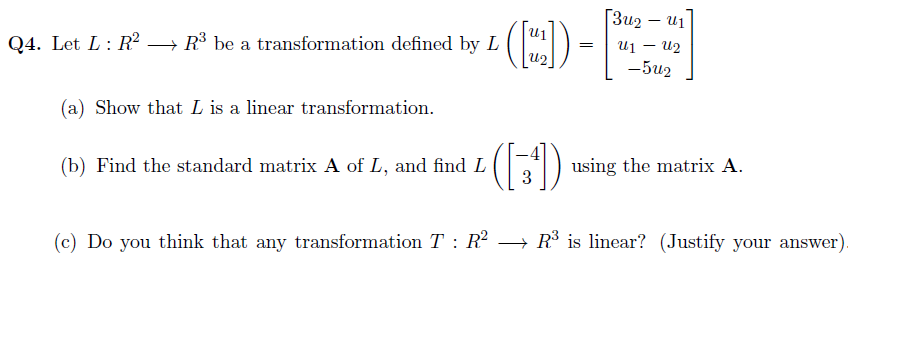Q4. Let L: R2 + Rº be a transformation defined by L (0-2 [3u2 – U1 U1 – U2 -502 (a) Show that I is a linear transformation. (b) Find the standard matrix A of L, and find L () using the matrix A. (c) Do you think that any transformation T:R2 + R² is linear? (Justify your answer).